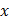# Loudness of sound from an isotropic point source at a distance at a distance of 70 cm is 20 dB. What is the distance (in m) at which it is not heard

## Question ID - 153938 :- Loudness of sound from an isotropic point source at a distance at a distance of 70 cm is 20 dB. What is the distance (in m) at which it is not heard

3537

 (7) Intensity from a point source varies with distance asLet at distancem, intensity isThen given 20(i) Let for, sound level be zero. Then intensity at that point should beand(ii) From Eqs. (i) and (ii), we getA point source of sound is located somewhere along the-axis. Experiments show that the same wave front simultaneously reaches listeners atm and. A third listener is positioned along the positive-axis. What is her- coordinate (in m) if the same wave front reaches her at the same instant as it does the first two listeners?